# Extraction of lineaments from gravity anomaly maps using the gradient calculation: Application to Central Anatolia

## Abstract

Linear anomalies are important in the interpretation of gravity and magnetic data because they indicate some important structural features. In this study, a method is proposed to extract lineaments which may be difficult to recognize. It is based on the convolution between the templates obtained from the second horizontal derivative of the theoretical anomaly due to a truncated horizontal plate and the gravity anomaly. Local maxima of the gradient magnitude determine lineaments in a gravity anomaly map. The method is used in the edge detection of geological structures closer to the surface, which are masked by other structures with various depths and dimensions. A source code in Matlab format for the proposed algorithm is implemented. Firstly, it was tested on synthetic examples and satisfactory results were obtained. Secondly, it was tested on gravity-anomaly maps of the Tuzgölü (Salt Lake) basin in Central Anatolia, Turkey. Subsequently, the proposed algorithm and normalized standard deviation (NSTD), another edge-detection filter, were evaluated together to obtain the horizontal location of potential-field source boundaries. A visual evaluation of the outputs was carried out and the results compared with each other. These examples demonstrate that the proposed method can be used in the visual interpretation of gravity-anomaly maps in order to detect geological features such as major and minor faults.

## 1. Introduction

One of the most common problems encountered in geophysical studies is how to determine the geometry of faults, or contacts, at different depths. Gravity is an effective geophysical method, for determining vertical geological features. These vertical discontinuities, which might correspond to subsurface faults and contacts, appear on gravity and magnetic maps as lineaments. Numerous techniques exist in geophysics to analyze gravity anomalies produced by faults or the boundary of two geologic zones. Major faults which can be defined as first-order faults can be inferred from gradient zones on gravity-anomaly maps (Zeng et al., 1994). But, it is generally difficult to recognize secondary faults which may be masked due to larger structures.

Edge-detection algorithms are suitable for the analysis of potential-field data. These are used in the visual interpretation of both gravity, and magnetic, maps in order to detect main geological structures. Lineaments in gravity and magnetic maps are used to identify areas of high priority for further detailed exploration. The Radon transform is a useful method for analyzing lineaments in a 2-D map. Pawlowski (1997) has shown the applicability of the Radon transform in displaying linear features in magnetic maps. Yunxuan (1992) applied the Radon transform to synthetic gravity maps in order to demonstrate its usefulness for upward and downward continuation to remove unwanted linear features. Sykes and Das (2000) used the Radon transform to enhance linear features in geophysical maps. Boschetti (2005) has proposed different edge-detection algorithms based on the use of horizontal or vertical gradients of potential-field anomalies. Aydogan (2007) interpreted lineaments of the Bouguer anomaly map of Biga, and its surrounding area, by using a cellular neural network algorithm that is used for image processing. Several edge-detection algorithms can be found in the literature, all of which are based on image gradients. These gradients are calculated from the image itself, rather than measured. Edge detection neither generates new information, nor extracts information from geophysical maps. It only transforms such information (Boschetti, 2005). Via edge detection, the information which is contained within the entire image pixel set is displayed in a form that helps the user to focus on the main features of a map and to interpret them in terms of geological structures (Hornby et al., 1999).

Works on edge detection have been adopted by various authors (Blakely and Simpson, 1986; McGrath, 1991; Mallat and Zhong, 1992; Moreau et al., 1997; Boschetti et al., 2001; Trompat et al., 2003; Cooper and Cowan, 2006). Several authors have developed various methods based on the use of horizontal, or vertical, gradients of potential-field anomalies (Rao et al., 1981; Grauch and Cordell, 1987; Reid et al., 1990; Marcotte et al., 1992; Roest et al., 1992; Zeng et al., 1994; Hsu et al., 1996; Fedi and Florio, 2001).

Since potential-field data correspond to the superposition of effects from all causative sources, the determination of geologic boundaries usually suffers from nearby source interference which produces false edges. In this work, our edge operator is based on the second horizontal derivative of a truncated horizontal-plate model. This approach is a useful tool for detecting deep faults, even in the presence of shallower near-surface features. The validity of the algorithm is proved by experiments on both synthetic and real examples. The real data in the study are from the Tuzgölü basin and surrounding area in Central Anatolia which has been the subject of numerous studies. Several earlier tectonic studies have been carried out for different purposes in the region (Arpat and Şaroglu, 1975; 0lkişik et al., 1997; CÇ emen et al., 1999; Gürer and Aldanmaz, 2002; Atesş et al., 2005; Büyüksaracç et al., 2005; Aydemir and Ates, 2006, 2008). Our approach is also confirmed for the popular method of normalised standard deviation used to estimate the horizontal location of potential-field source boundaries (Cooper and Cowan, 2008). This method, as applied to the location of edges of causative sources, is simple and easy to use and is a good approach for determining vertical, or near vertical, faults.

## 2. Lineament Extraction and Mapping

In image processing, edge detection is a process which attempts to capture significant features from the image. The main idea of edge-detection techniques is to locate the geological features for gravity-data processing and interpretation. One may consider the input of the edge detector as a discrete image of gravity data, and its output as another image called the gravity edge map. It is difficult to design a general edge-detection algorithm which performs well in many contexts. Canny (1986) was the first to give analytic expressions for the criteria that have to be optimized in edge detection. In the case of a low noise level, gradient estimations are the classical methods which give satisfactory results. Lunscher (1983), Modestino and Fries (1977), and Shanmugam and Green (1979) have developed different edge-detection operators by associating low-pass filtering with the gradient detector. Shen and Castan (1986) proposed an algorithm based on a recursive form with exponential impulse response to detect step edge using a second-derivative filter.

In gravity interpretation, the edges come in many forms and shapes. They might be in the form of faults, contacts, and various structural boundaries. The gradient extreme of anomalies usually correspond to the boundaries of the existence of these anomalies and have been used to approximate edges of source bodies or to analyze lineaments (Blakely and Simpson, 1986). In order to highlight lineaments of gravity data, we make use of a truncated horizontal-plate model. The truncated horizontal-plate model is a popular structure for geophysical studies. This model, shown in xz cross-section in Fig. 1(d), semi-infinite in the positive x-axis direction, is bounded by upper and lower horizontal planes z1 and z2. Assuming uniform density distributions in the model and the surrounding medium, the gravity effect, and the first and second horizontal derivatives, are given by, respectively,(1)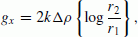(2)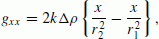(3)

(Telford et al., 1976). Where k is the gravitational constant, and Δ ρ is the density contrast between the model and the surrounding medium,

Many filters are available to enhance subtle detail in potential-field data, such as downward continuation, horizontal and vertical derivatives, and other forms of highpass filters. A commonly-used edge-detection filter is the total horizontal derivative (Cooper and Cowan, 2008). It can be used to enhance certain features, smooth out noise or highlight previously-known shape in potential-field data. The horizontal gradients can be estimated from the convolution kernels (referred to as gradient operator, edge detector, mask operator and template). The two-dimensional cross-correlation is a simple and robust algorithm used to solve different problems in the field of image processing. In the space domain, if L is the gradient operator (n×m) and g(x, y) is the potential-field data (M × N), cross-correlation of L with g is written as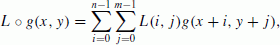(4)

where the circle denotes cross-correlation. The mathematical definition of discrete convolution is written as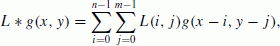(5)

where the asterisk denotes convolution. Equations (4) and (5) are identical when the gradient operator is symmetric. The key difference between the two is that convolution is associative. However, in the frequency domain we can express it as a multiplication of the Fourier transforms of the given functions.

The first step in image processing is the estimation of the derivatives in the x- and y-directions in order to smooth the image. In the time domain, this process is carried out through the convolution of the 2-D Gaussian function and the image using the following equations.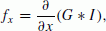(6a)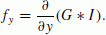(6b)

Here, I is the image, G is the 2-D Gaussian function, f x and f y are the derivatives in the x- and y-directions, respectively. These expressions are notso effectivein gradient-calculations. Using the properties of the convolution process in a more effective way is given below (Canny, 1986);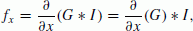(7a)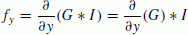(7b)

The magnitude of the gradient is given by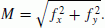(8)

In this study, the first horizontal derivative of the truncated horizontal-plate model equation (Eq. (2)) was used to smooth the gravity data. Here, g is the gravity data, g x and g y are the first horizontal derivatives in the x- and y-directions which can be estimated from the Eqs. (9) and (10).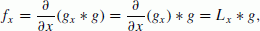(9)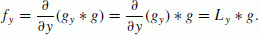(10)

Here, L x and L y are the mask operators in the x- and y-directions derived from Eq. (3), respectively. Also, we have used the second horizontal derivative expression (Eq. (3)) in order to estimate the coefficients of the mask operators. These are shown in Fig. 2.

The edge can be identified by the closed maximum of convolution between these kernels and the gravity data. For the 1-D example, gravity-anomaly (Fig. 1(a)) and edge-operator coefficients (Fig. 1(b)) have been computed along the profile using Eqs. (1) and (3). Figure 1(c) displays the convolution output. As shown in Fig. 1(c), the maximum amplitude of the convolution output delineates the edge of this model quite well.

The edge-detection process can be considered as a pixel classification process by the determination of some pixel as a boundary. It can be determined whether the pixel is a boundary pixel, or not, by taking into account the pixels and its neighboring values for each pixel in a digitized image.

In the image process, a threshold value is generally applied to gradient magnitude values in order to determine lineaments of an image. The threshold value is the most significant parameter that is used in the stage of determining edges from a filtered image. A number of studies in the literature are available for selecting the threshold value in image processing problems: some of which are Prewitt and Mendelsohn (1966), Otsu (1979), Pun (1980) and Tsai (1985).

After gravity data are transformed into the gradient domain, a threshold can be given, and the gradient magnitude whose corresponding gradient values are greater than the threshold can then be obtained. These maxima indicate the strike of lineaments. This is the lineaments identification procedure. Enhancement, or attenuation, of certain lineaments can be realized through non-maximum suppression. The edge map is obtained by taking the gradient of the input data and limiting it to an available threshold value. All values below the threshold are set to zero. Finally, an edge map is obtained. However, it is difficult to choose a proper threshold. Because of this difficulty, in the edge map there may still be false edges if the threshold is too low or some edges may be missing if the threshold is too high (Peli and Malah, 1982; Hertz and Schafer, 1988; Chen and Shrestha, 2000; Sun et al., 2007; Chen and Ho, 2008). A double threshold can be applied to overcome this problem. Local maxima of the gradient magnitude determine edges in the input data. It is evident that a gradient-based edge detector is an effective and practical approach for both gravity and magnetic data interpretation. This approach can be used to extract geological features such as faults and contacts from a gravity-anomaly map.

## 3. Synthetic Examples

In this section, 2-D synthetic examples are used to test the effectiveness of the proposed approach. Some practical results are presented and briefly discussed to demonstrate the success of this approach. Lineaments in the gravity map are indicative of contacts, basement faulting, and other tectonic features of interest. Our goal is to detect edges of geological features which are the cause of lineaments in the gravity map. In this context, an appropriate edge operator is proposed for the determination of lineaments.

Synthetic Model (1), shown in Fig. 3(a), is produced by using finite rectangular vertical prisms located at various depths. The top and bottom depths of prism A were selected as 2 and 5 km, the widths of prism A in the x and y directions were selected as 30 and 40 km, respectively. Prism B is deeper than prism A. The top and bottom depths of prism B were selected as 5 and 10 km, and the widths of prism B in the x and y directions were selected as 20 and 40 km, respectively.

The synthetic gravity-anomaly map is shown in Fig. 3(a). In the present study, it is assumed that 1 pixel corresponds to 1 km. The model considers 101 × 101 pixels in area. The proposed algorithm is tested on a gravity-anomaly map of synthetic Model (1). Using Eq. (3), we obtained 5 × 5 masks, shown in Fig. 2, for calculating the derivatives in the x- and y-directions. After the gradients of the gravity data in x- and y-directions are calculated from Eqs. (9) and (10), a gradient magnitude map is obtained from Eq. (8). After that, non-maxima suppression is performed and an edge map is obtained. For the non-maxima suppression, the combinations of high and low threshold values (Th and T1, respectively) were selected based on trial-and-error experiments. We have found that Th = 0.4 and T1 = 0.1 produce the best gravity edge map. As demonstrated in Fig. 3(c), the method delineates the edges of the prisms quite well.

To obtain adequate information from the filtered output, a threshold operation can be performed. By designating a threshold value, an indented level of detail can be obtained. The applied threshold value could be important in a location whose structure range extends from a narrow fracture to a broad valley. The classification of structures can be easily made by taking an accurate threshold value. In the compilation of a tectonic map, an assortment of lineaments is applied. The gradient of the slope and the magnitude of the value are comparative in the filtered image. Lineaments are described by an appropriate level of pixel values. In general, a threshold value can be designated in two ways. One way is that it can be determined from a few known structures in the area or from an available tectonic map. It may also be determined by using professional opinion. To some extent, this latter way introduces subjectivity into the mapping process.

If the lower threshold is set too high, it will induce noisy edges to break up. If the upper threshold is set too low, it will lead to many artificial and unwanted edge fragments that are seen in the output (Fig. 5(a–c)). When a higher threshold is employed, brighter areas contain less false edges, but many edges are missed in darker areas (Fig. 5(d)). The threshold method may help to obtain data from the filtered output. In order to obtain desired details, the threshold values can be adjusted accordingly. The effect of the threshold value on the output image is shown in Fig. 5(a–d).

To compare the proposed approach with classical derivative techniques, a NSTD method is applied on the same gravity-anomaly map. This method is based on the ratio of related normalized standard deviations (NSTD) to make large and small amplitude edges visible simultaneously (Cooper and Cowan, 2008). An NSTD map of the gravity data is shown in Fig. 3(b).

To demonstrate how this approach performs on noisy data, random noise with an amplitude equal to 0.07% of the maximum data amplitude was added to the gravity data set shown in Fig. 3(a). Figures 4(a), 4(b) and 4(c) show, respectively, the anomaly map with added noise, the NSTD obtained from the noisy anomaly map and the outputs of the proposed method. In the case of noisy data, it is seen that the proposed method produces better results than the NSTD method (Fig. 4(b)).

The performance of the method in terms of different threshold values for the theoretical model shown in Fig. 3(a) has been investigated and the results are shown in Fig. 5.

In the case of selecting a high threshold value, the effects with long wavelength of the prism B could not be observed (Fig. 5(d)).

For the determination of geologic boundaries, a more sophisticated example (Model 2) is shown in Fig. 6(a). This model is formed by three rectangular prisms which are located at various depths and dimensions. The prismatic blocks constructing the model are located at depths A, B and C, given in increasing depth order. The geometric positions of A and B prisms are the same as the theoretical model shown in Fig. 4(a). The top and bottom depths of prism C were selected as 4 and 7 km, and the widths of C prism in the x- and y-directions were taken as 12 and 14 km, respectively.

The model is 101 × 101 pixels in area. The synthetic gravity-anomaly map is shown in Fig. 6(a). The same procedures used for Model (1) are repeated to locate the edges of causative sources. The NSTD map is shown in Fig. 6(b). The results obtained using the proposed method is given in Fig. 6(c). As we did for the first synthetic model, we add noise to the data of the second model (Fig. 7(a)) and applied both the NSTD method, and the proposed method, so as to determine the lineaments, yielding the results given in Figs. 7(b) and 7(c), respectively. In the case of noisy data, we obtained poor resolution for the borders of the B and C prisms. The effect of the threshold value on the output image is shown in Fig. 8(a–d). It is observed that with high threshold values, the effects with a long wavelength in the theoretical models are completely lost, while erroneous lineaments are produced with low threshold values.

Experimental results demonstrate that our operator, with the use of appropriate threshold values, may produce an edge map from input data. It is shown that the proposed edge-detection algorithm produced fairly good results on both simple and more complex synthetic examples. The performance of the proposed method is quite good. Threshold values play an important role in the identification of lineaments. In terms of the estimation power and the computation time, the method can be considered as an effective and powerful tool in the interpretation of gravity and magnetic data.

## 4. Application to Real Data

Part of the Tuzgölü basin and its surrounding area in Central Anatolia, Turkey, was selected as a study area to demonstrate the capabilities of the proposed algorithm for lineament detection.

A simplified tectonic map of the Tuzgölü basin (compiled from Çemen et al., 1999) is shown in Fig. 9. A northwest-striking fault zone is the most prominent structural feature along the eastern margin of the Tuzgölü basin. This structural feature was named the Tuzgölü fault zone by Arpat and Şaroglu (1975). It was also referred to as the Kochisar-Aksaray fault (Görür et al., 1984) and as the Aksaray-S. Kochisar fault (Şengör et al., 1985). In the western part of the basin, two parallel fault zones extend from northwest of Yeniceoba and Cihanbeyli. The northernmost of the two faults is called the Yeniceoba fault zone and the southern one is named the Cihanbeyli fault zone. The Yeniceoba fault zone has been displaced by a northeast striking fault and probably connects to the north- to northwest-striking Sultanhani fault zone. To test the ability of the algorithm to detect major and minor faults, the lineaments are compared with the faults shown in Fig. 9.

A Bouguer gravity-anomaly map of the study area (compiled from (Uğurtaş, 1975) is shown in Fig. 10. The gravity anomaly map, which is the main geophysical data used to identify faults, is digitized at 1 km intervals. The gradient components of the input data in the x- and y-directions are calculated from Eqs. (9) and (10), respectively. In Eq. (8), these components are used to compute the gradient magnitude of the input data (Fig. 10). Once the gradient magnitude of the input data has been calculated, non-maxima suppression is performed. The information outside the body edges is eliminated by applying threshold values. A better edge map is obtained when Th = 0.4 and T1 = 0.1 (Fig. 11(a)). It is observed from this map that the proposed approach can detect major and minor lineaments, but some false lineaments are also detected. The output map is simpler and easier to recognize.

The tectonic map, the Bouguer anomaly map, and the NSTD map, which allow us to detect geologic boundaries of causative sources, are integrated to provide evidence of the determination of faults. In the study area, 7 faults are identified after merging 25 lineaments into single faults. Figure 11(a) shows lineaments superimposed on the Bouguer anomaly map. Lineaments are detected and extracted by the proposed algorithm from Fig. 10. Figure 11(b) provides auxiliary information for fault interpretation. Most of the geological features can be visually shown to fit in with our results. According to defined fault systems, the L1–L3 lineaments which appeared in the gravity-anomaly map as a gradient zone geologically belong to one fault system (F1) when they are combined (Fig. 11(a)). This fault is not seen in Fig. 11(b) as visually. The L4–L11 lineaments are considered to be one fault system (F2) after they are combined. This fault is consistent with Fig. 11(b) and it also overlaps with the buried fault seen in Fig. 9. The fault system (F3) that is obtained by combining the L12–L16 lineaments is compatible with the buried fault system extending in the NW-SE direction to the west of Sultanhani. It could be said that the L17–L18 lineaments coincide with the normal fault (F4) located at the eastern side of Tuzgölü. This fault is also supported by Fig. 11(b). By combining the L19–L22 lineaments which are formed in the same gradient zone, it is observed that they represent the strike-slip Tuzgölü fault (F5). The L23 lineament can be considered as a single fault system (F6) oriented in the N-S direction in the gradient zone, and L23 is compatible with the buried fault shown in Fig. 9. It could be said that the L24 and L25 lineaments create one fault system (F7) when they are combined and this fault is supported by Fig. 11(b). Here identified fault traces are named F1–F7 and are shown on Fig. 11(a) as straight lines. According to the NSTD method, our algorithm shows more similarity to the tectonic map displayed in Fig. 9. The results of a comparison with the tectonic map show that our proposed algorithm is able to detect more of the faults shown in Fig. 9 than the number identified from the visual interpretation of geologists. In the southwestern part of the study area, several minor lineaments which are not to be seen on the tectonic map are shown determined on the map obtained by the proposed algorithm. The relationship between these lineaments and faults can be identified from detailed geophysical studies. Lineaments are extracted as a steep gradient of a gravity anomaly, which corresponds to a density contrast at depth. Faulting is, of course, a possible cause of the density contrast, but other explanations (erosion, etc.) may also be valid. So it is definitely necessary to carry out an additional field survey, or geophysical exploration, to check the result (Takeshi Sagiya, personal communication, 2011).

The proposed method is a tool to aid geophysical interpretation. However, the role of interpreters explaining the geological importance of results remains very important.

## 5. Conclusions

In this study, an edge operator based on the second horizontal derivative of a truncated horizontal-plate model is used to process gravity data and to realize the detection and extraction of lineaments. We have applied a different gradient-interpretation algorithm for lineament mapping purposes. The application of the proposed method in gravity mapping is not common. As part of the detection procedure, a threshold-based gradient method is used to highlight long linear segments or to enhance short linear ones. It is evident that a gradient-based edge detector is an effective and practical approach for both gravity and magnetic interpretations. Searching for discontinuities in the input data corresponds to searching for the local maxima of the gradient magnitudes. These maxima provide the edge information of the input data.

Firstly, the proposed edge operator has been applied on two sets of synthetic data in order to detect borders of vertical prismatic blocks and the results are compared with those of another edge detection filter (NSTD) (Figs. 38). After obtaining satisfactory results, the method was applied to the Gravity anomaly map of the Tuzgölü basin in Central Anatolia. The structural features in the area between Yeniceoba, Cihanbeyli, S. Kochisar, Aksaray and Sultan-hani were investigated. The results of the proposed approach (Fig. 10(a)) show a good agreement with previously-observed faults in the field (CÇ emen et al., 1999; Aydemir and Ates, 2006, 2008). Faults that are not clear in the gravity anomaly map can also be identified as well. These results show that the proposed approach constitutes a useful tool to aid fault interpretation. It is observed from the experimental results that increasing the operator size and the threshold values reduce its sensitivity to noise, at the expense of losing some of the real edges in the input data. The threshold value is a key parameter in the proposed approach (Figs. 5 and 8). A selection of the threshold value from the gradient magnitude is related to the accuracy of lineament detection. The localization error in the detected edges also increases as the operator size and threshold value are increased. In other words, it is a way to keep the significant information of the input data whilst getting rid of the unimportant parts. The experimental results demonstrate that this operator can be used in the visual interpretation of gravity-anomaly maps in order to detect geological features such as major and minor faults.

Consequently, by applying the proposed approach to a gravity-anomaly map, a detailed general view of surface and subsurface structural boundaries can be obtained successfully. The proposed approach, as applied to the location of edges of causative sources, is simple and easy to use, and is good for vertical or near vertical faults. The method is a tool that can contribute to the geophysical interpretation in determining fault traces, but the observations of experienced reviewers are needed to define the geological meaning of the results. Thus, this method should be considered to be a useful tool, like other geophysics techniques, in the investigation of structural boundaries over wide areas.

## References

1. Arpat, E. and F. Şaroglu, Türkiye’deki bazi önemli genç tektonik olaylar, Turkish Geol. Soc. Bull., 18, 91–101, 1975.

2. Atesş, A., F. Bilim, and A. Büyüksaracç, Curie Point Depth investigation of Central Anatolia, Turkey, Pure Appl. Geophys., 162, 357–371, 2005.

3. Aydemir, A. and A. Ates, Structural interpretation of the Tuzgölü and Haymana Basins, Central Anatolia, Turkey, using seismic, gravity and aeromagnetic data, Earth Planets Space, 58, 951–961, 2006.

4. Aydemir, A. and A. Ates, Determination of hydrocarbon prospective areas in the Tuzgolu (Salt lake) Basin, central Anatolia, by using geophysical data, J. Petrol. Sci. Eng., 62, 36–44, 2008.

5. Aydogan, D., Processing the Bouguer anomaly map of Biga and the surrounding area by the Cellular Neural Network: Application to the southwestern Marmara region, Earth Planets Space, 59, 201–208, 2007.

6. Blakely, R. J. and R. W. Simpson, Approximating edges of source bodies from magnetic or gravity anomalies, Geophysics, 51, 1494–1498, 1986.

7. Boschetti, F., Improved edge detection and noise removal in gravity maps via the use of gravity gradients, J. Appl. Geophys., 57, 213–225, 2005.

8. Boschetti, F., P. Hornby, and F. G. Horowitz, Wavelet based inversion of gravity data, Exp. Geophys., 32,48–55, 2001.

9. Büyüksaracç, A., D. Jordanova, A. Atesş, and V. Karloukovski, Interpretation of the gravity and magnetic anomalies of the Cappadocia region, central Turkey, Pure Appl. Geophys., 162, 2197–2213, 2005.

10. Canny, J., A computational approach to edge detection, IEEE Transaction on Pattern Analysis and Machine Intelligence, PAMI 8 (6), 679–698, 1986.

11. Çemen, I., M. C. Göncüoglu, and K. Dirik, Structural evolution of the Tuzgölü basin in Central Anatolia, Turkey, J. Geol., 107, 693–706, 1999.

12. Chen, C. H. and P. G. Ho, Statistical pattern recognition in remote sensing, Pattern Recognition, 41, 2731–2741, 2008.

13. Chen, C. H. and B. Shrestha, Classification of multi-sensor remote sensing images using self-organizing feature maps and radial basis function networks, in Proceedings of International Geosciences and Remote Sensing Symposium (IGARSS), Hawaii, 2000.

14. Cooper, G. R. J. and D. R. Cowan, Enhancing potential field data using filters based on the local phase, Comput. Geosci., 32, 1585–1591, 2006.

15. Cooper, G. R. J. and D. R. Cowan, Edge enhancement of potential-field data using normalized statistics, Geophysics, 73, H1–H4, 2008.

16. Fedi, M. and G. Florio, Detection of potential fields source boundaries by enhanced horizontal derivative method, Geophys. Prospect., 49,40–58, 2001.

17. Görür, N., F. Y. Oktay, I. Seymen, and A. M. C. Şengör, Paleotectonic evolution of Tuzgölü basin complex, Central Turkey, in The Geological Evolution of the Eastern Mediterranean, edited by Dixon, J. E. and A. H. F Robertson, Spec. Publ. Geol. Soc., 17, 81–96, 1984.

18. Grauch, V. J. S. and L. Cordell, Limitations of determining density or magnetic boundaries from the horizontal gradient of gravity or pseudogravity data, Geophysics, 52, 118–121, 1987.

19. Gürer, Ö. F. and E. Aldanmaz, Origin of the Upper Cretaceous-Tertiary sedimentary basins within the Taurid-Anatolide platform in Turkey, Geol. Mag., 139 (2), 191–197, 2002.

20. Hertz, L. and R. W. Schafer, Multilevel thresholding using edge matching, Comput. Vision Graphics Image Process., 44, 279–295, 1988.

21. Hornby, P., F. Boschetti, and F. Horowitz, Analysis of potential field data in the wavelet domain, Geophys. J. Int., 137, 175–196, 1999.

22. Hsu, S. K, J. C. Sibuet, and C. T. Shyu, High-resolution detection of geologic boundaries from potential field anomalies: an enhanced analytic signal technique, Geophysics, 61, 373–386, 1996.

23. İlkişik, O. M., A. Gürer, T. Tokgöz, and C. Kaya, Geoelectromagnetic and geothermic investigations in the Ihlara Valley geothermal field, J. Volcanol. Geotherm. Res., 78, 297–308, 1997.

24. Lunscher, W. H. H. J., The asymptotic optimal frequency domain filter for edge detection, IEEE Transaction on Pattern Analysis and Machine Intelligence, PAMI 5 (6), 678–679, 1983.

25. Mallat, S. and S. Zhong, Characterization of signals from multiscale edges, IEEE Transactions on Pattern Recognition and Machine Intelligence, 14, 710–732, 1992.

26. Marcotte, D. L., C. D. Hardwick, and J. B. Nelson, Automated interpretation of horizontal magnetic gradient profile data, Geophysics, 57, 288–295, 1992.

27. McGrath, P. H., Dip and depth extent of density boundaries using horizontal derivatives of upward continued gravity data, Geophysics, 56, 1533–1542, 1991.

28. Modestino, J. W. and R. W. Fries, Edge detection in noisy images using recursive digital filter, Comput. Graphics Image Process, PAMI 1, 409–433, 1977.

29. Moreau, F., D. Gibert, M. Holschneider, and G. Saracco, Wavelet analysis of potential fields, Inverse Problems, 13, 165–78, 1997.

30. Otsu, N., A threshold selection method from gray-level histograms, IEEE Transactions on Systems, 9(1), 62–66, 1979.

31. Pawlowski, R. S., Use of slant stack for geologic or geophysical map lineament analysis, Geophysics, 62, 1774–1778, 1997.

32. Peli, T. and D. Malah, A study of edge detection algorithms, Comput. Graphics Image Process., 20,1–21, 1982.

33. Prewitt, J. M. S. and M. L. Mendelsohn, The analysis of cell images, Ann. Newyork Academy Sci., 128, 1035–1053, 1966.

34. Pun, T., A new method for gray-level picture thresholding using the entropy of the histogram, Signal Processing, 2, 223–237, 1980.

35. Rao, D. A., H. V. Babu, and P. V. Narayan, Interpretation of magnetic anomalies due to dikes: The complex gradient method, Geophysics, 46, 1572–1578, 1981.

36. Reid, A. B., J. M. Allsop, H. Granser, A. J. Millett, and I. W. Somerton, Magnetic interpretation in three dimensions using Euler deconvolution, Geophysics, 55,80–91, 1990.

37. Roest, W. R., J. Verhoef, and M. Pilkington, Magnetic interpretation using the 3-D analytic signal, Geophysics, 57, 116–125, 1992.

38. Şengör, A. M. C., N. Görür, and F. Şaroglu, Strike slip deformation basin formation and sedimentation, strike-slip faulting and related basin formation in zones of tectonic escape, Turkey as a case study, in Strike-slip Faulting and Basin Formation, Soc. Econ. Paleont. Mineral. Spec. Pub., edited by Biddle, K. T. and N. Christie-Blick, 37, 227–264, 1985.

39. Shanmugam, K. S. and J. A. Green, An optimal frequency domain filter for edge detection in digital pictures, IEEE Transaction on Pattern Analysis and Machine Intelligence, 1, 37–49, 1979.

40. Shen, J. and C. Castan, An optimal linear operator for edge detection, Proceeding Conference on Vision and Pattern Recognition (CUPR), 109–114, 1986.

41. Sun, G., Q. Liu, C. Ji, and X. Li, A novel approach for edge detection based on the theory of universal gravity, Pattern Recognition, 40, 2766–2775, 2007.

42. Sykes, M. P. and U. C. Das, Directional filtering for linear feature enhancement in geophysical maps, Geophysics, 65, 1758–1768, 2000.

43. Telford, W. M., L. P. Geldart, R. E. Sheriff, and D. A. Keys, Applied Geophysics, Cambridge University Press, 1976.

44. Trompat, H., F. Boschetti, and P. Hornby, Improved downward continuation of potential field data, Expl. Geophys., 34 (4), 249–256, 2003.

45. Tsai, W., Moment preserving thresholding: A new approach, Comput. Vision Graphics Image Processing, 29, 377–393, 1985.

46. Uğurtaş, G., Geophysical interpretation of part of the Tuzgölü basin, M. T. A. Bull., 85,38–45, 1975.

47. Yunxuan, Z., Application of the Radon transform to the processing of airborne geophysical data, Ph.D. thesis, Internat, Inst., Aerospace Survey and Earth Sciences, 1992.

48. Zeng, H., Q. Zhang, and J. Liu, Location of secondary faults from cross-correlation of the second vertical derivative of gravity anomalies, Geophys. Prospect., 42, 841–854, 1994.

## Acknowledgments

The author thanks Dr. Aysan Gürer, Dr. Ali Pinar, Dr. Ali Elmas and Dr. Nihan Sezgin for their careful reading and suggestions to improve the manuscript. I would also like to thank an anonymous referee and Dr. Takeshi Sagiya for their reading and constructive comments on the manuscript.

## Author information

Authors

### Corresponding author

Correspondence to D. Aydogan.

## Rights and permissions

Reprints and Permissions

Aydogan, D. Extraction of lineaments from gravity anomaly maps using the gradient calculation: Application to Central Anatolia. Earth Planet Sp 63, 903–913 (2011). https://doi.org/10.5047/eps.2011.04.003

• Revised:

• Accepted:

• Published:

• Issue Date:

### Key words

• Gravity anomaly